Скачать презентацию Making paper cups What you ll need 1 Paper

f56a5d75c6d3b3d5089b5ea2bcbd3766.ppt

• Количество слайдов: 30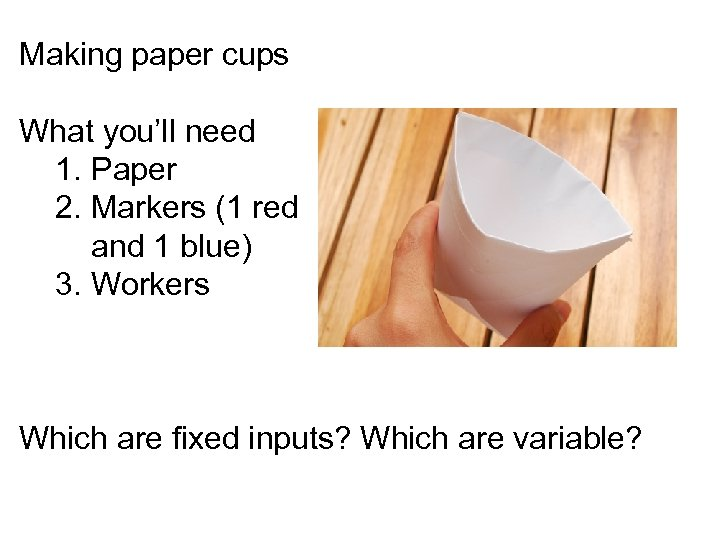Making paper cups What you’ll need 1. Paper 2. Markers (1 red and 1 blue) 3. Workers Which are fixed inputs? Which are variable?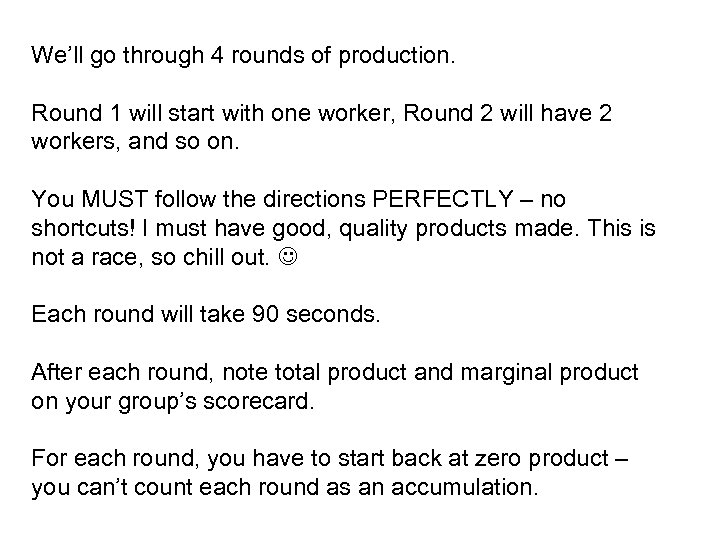We’ll go through 4 rounds of production. Round 1 will start with one worker, Round 2 will have 2 workers, and so on. You MUST follow the directions PERFECTLY – no shortcuts! I must have good, quality products made. This is not a race, so chill out. Each round will take 90 seconds. After each round, note total product and marginal product on your group’s scorecard. For each round, you have to start back at zero product – you can’t count each round as an accumulation.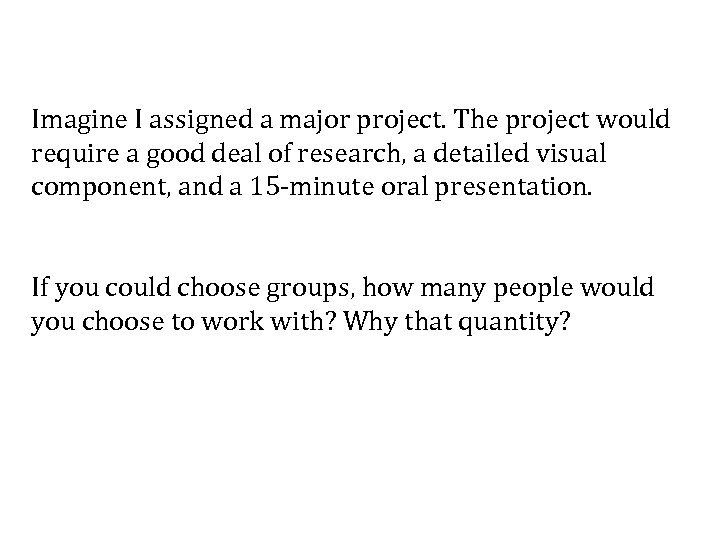Imagine I assigned a major project. The project would require a good deal of research, a detailed visual component, and a 15 -minute oral presentation. If you could choose groups, how many people would you choose to work with? Why that quantity?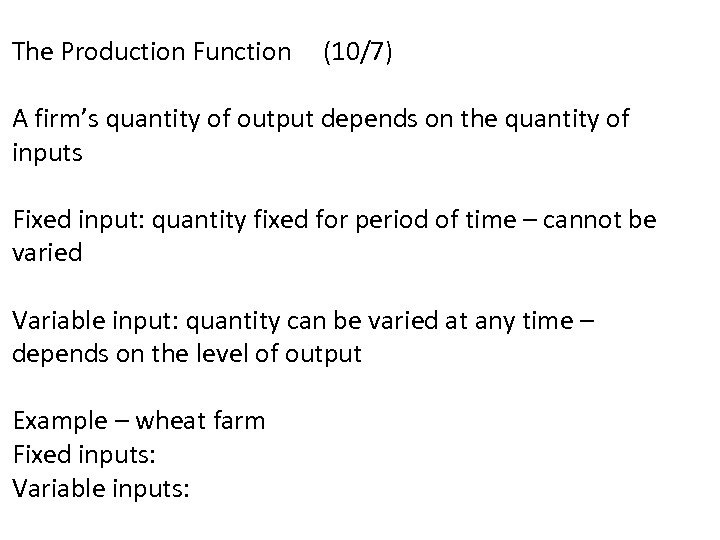The Production Function (10/7) A firm’s quantity of output depends on the quantity of inputs Fixed input: quantity fixed for period of time – cannot be varied Variable input: quantity can be varied at any time – depends on the level of output Example – wheat farm Fixed inputs: Variable inputs: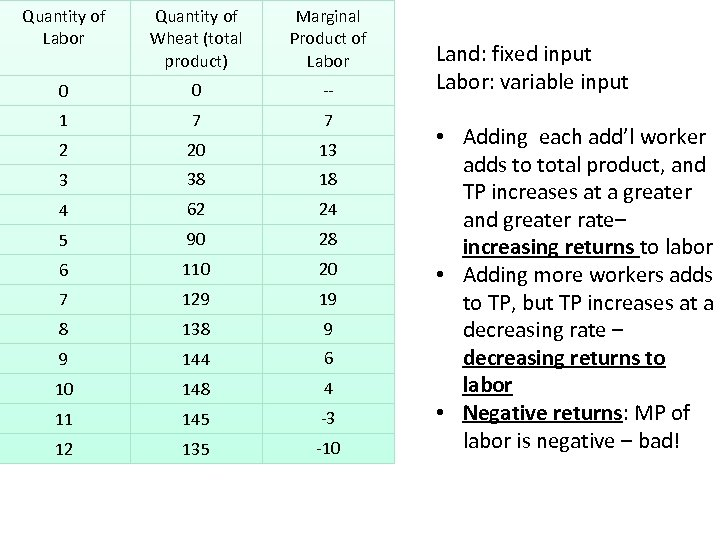Quantity of Labor Quantity of Wheat (total product) Marginal Product of Labor 0 0 -- 1 7 7 2 20 13 3 38 18 4 62 24 5 90 28 6 110 20 7 129 19 8 138 9 9 144 6 10 148 4 11 145 -3 12 135 -10 Land: fixed input Labor: variable input • Adding each add’l worker adds to total product, and TP increases at a greater and greater rate– increasing returns to labor • Adding more workers adds to TP, but TP increases at a decreasing rate – decreasing returns to labor • Negative returns: MP of labor is negative – bad!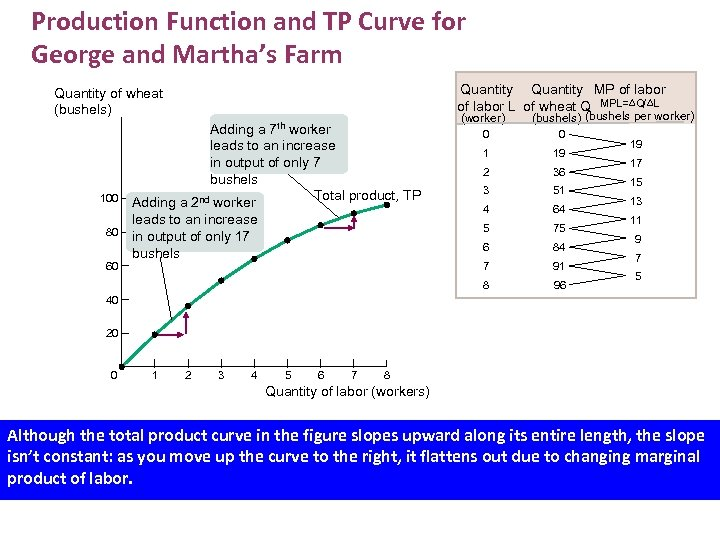Production Function and TP Curve for George and Martha’s Farm Quantity MP of labor L of wheat Q MPL= Q/ L Quantity of wheat (bushels) Adding a 7 th worker leads to an increase in output of only 7 bushels Total product, TP Adding a 2 nd worker leads to an increase in output of only 17 bushels (worker) (bushels per worker) 80 60 0 1 19 2 36 3 51 4 64 5 75 6 84 7 91 8 100 0 96 19 17 15 13 11 9 7 5 40 20 0 1 2 3 4 5 6 7 8 Quantity of labor (workers) Although the total product curve in the figure slopes upward along its entire length, the slope isn’t constant: as you move up the curve to the right, it flattens out due to changing marginal product of labor.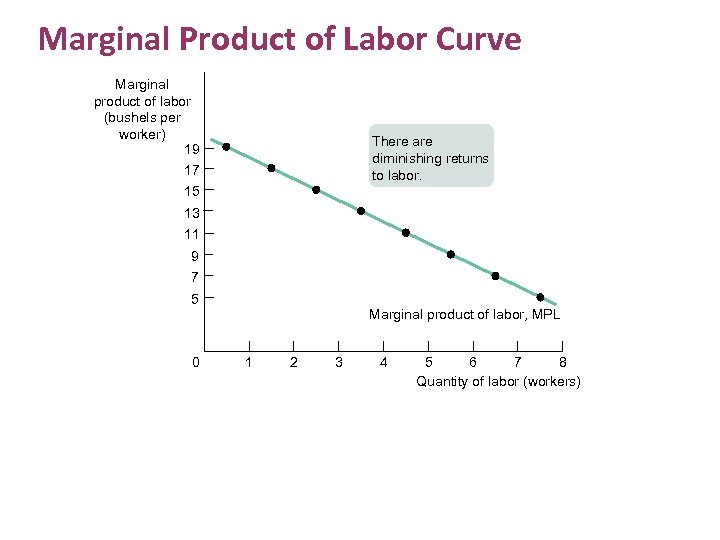Marginal Product of Labor Curve Marginal product of labor (bushels per worker) 19 There are diminishing returns to labor. 17 15 13 11 9 7 5 0 Marginal product of labor, MPL 1 2 3 4 5 6 7 8 Quantity of labor (workers)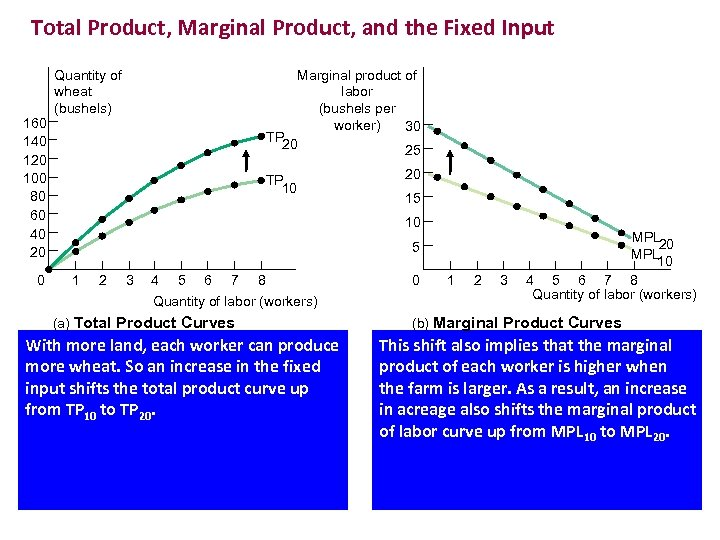Total Product, Marginal Product, and the Fixed Input 160 140 120 100 80 60 40 20 0 Quantity of wheat (bushels) Marginal product of labor (bushels per worker) 30 TP 20 25 TP 10 20 15 10 5 1 2 3 4 5 6 7 8 Quantity of labor (workers) (a) Total Product Curves With more land, each worker can produce more wheat. So an increase in the fixed input shifts the total product curve up from TP 10 to TP 20. 0 1 2 3 MPL 20 MPL 10 4 5 6 7 8 Quantity of labor (workers) (b) Marginal Product Curves This shift also implies that the marginal product of each worker is higher when the farm is larger. As a result, an increase in acreage also shifts the marginal product of labor curve up from MPL 10 to MPL 20.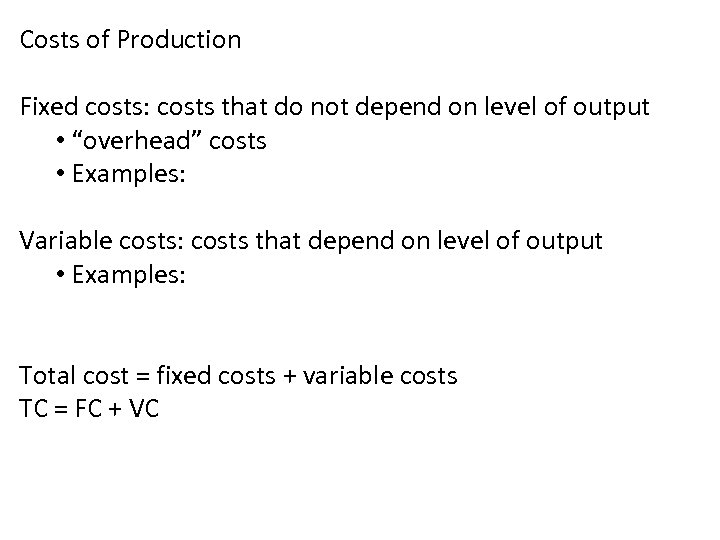Costs of Production Fixed costs: costs that do not depend on level of output • “overhead” costs • Examples: Variable costs: costs that depend on level of output • Examples: Total cost = fixed costs + variable costs TC = FC + VC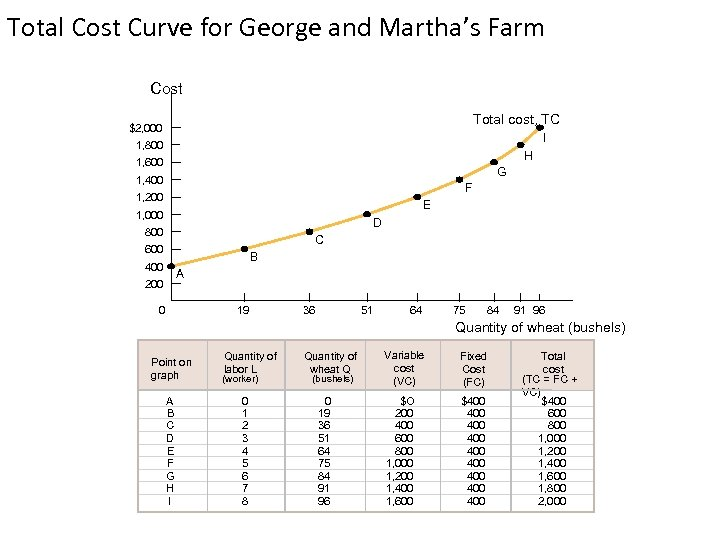Total Cost Curve for George and Martha’s Farm Cost Total cost, TC \$2, 000 I 1, 800 H 1, 600 G 1, 400 F 1, 200 E 1, 000 D 800 C 600 B 400 A 200 0 19 36 51 64 75 84 91 96 Quantity of wheat (bushels) Point on graph A B C D E F G H I Quantity of labor L (worker) 0 1 2 3 4 5 6 7 8 Quantity of wheat Q (bushels) 0 19 36 51 64 75 84 91 96 Variable cost (VC) Fixed Cost (FC) \$O 200 400 600 800 1, 000 1, 200 1, 400 1, 600 \$400 400 400 Total cost (TC = FC + VC) \$ 400 600 800 1, 000 1, 200 1, 400 1, 600 1, 800 2, 000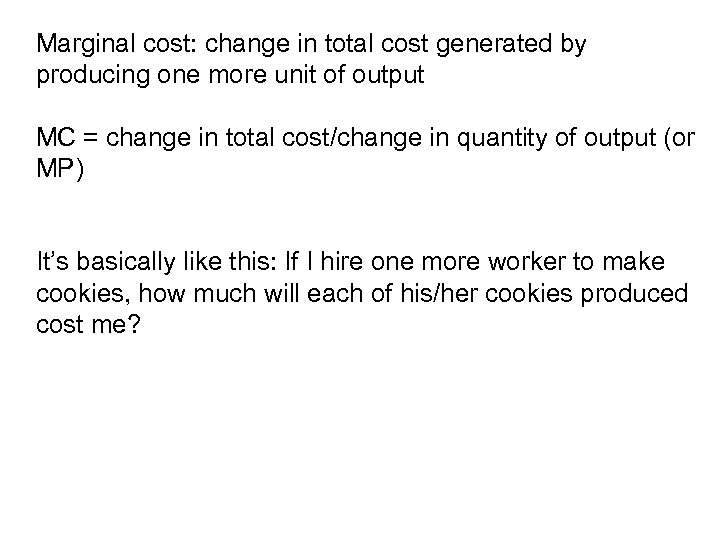Marginal cost: change in total cost generated by producing one more unit of output MC = change in total cost/change in quantity of output (or MP) It’s basically like this: If I hire one more worker to make cookies, how much will each of his/her cookies produced cost me?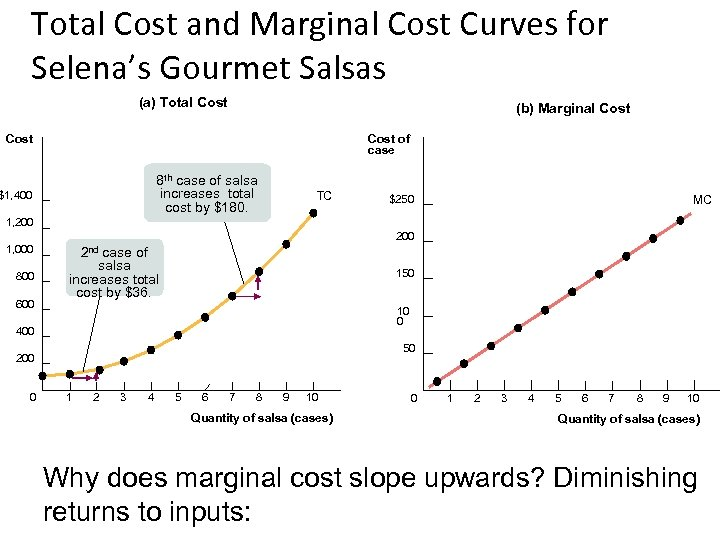Total Cost and Marginal Cost Curves for Selena’s Gourmet Salsas (a) Total Cost (b) Marginal Cost of case 8 th case of salsa increases total cost by \$180. \$1, 400 TC \$250 MC 1, 200 1, 000 800 600 2 nd case of salsa increases total cost by \$36. 150 10 0 400 50 200 0 1 2 3 4 5 6 7 8 9 10 Quantity of salsa (cases) Why does marginal cost slope upwards? Diminishing returns to inputs: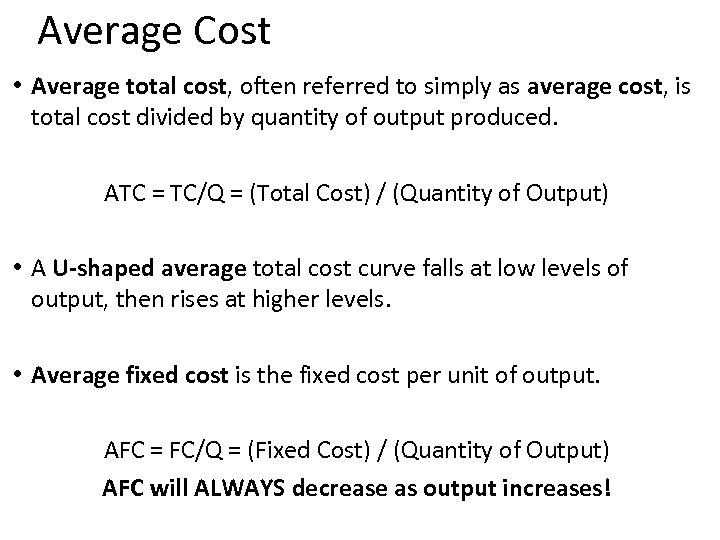Average Cost • Average total cost, often referred to simply as average cost, is total cost divided by quantity of output produced. ATC = TC/Q = (Total Cost) / (Quantity of Output) • A U-shaped average total cost curve falls at low levels of output, then rises at higher levels. • Average fixed cost is the fixed cost per unit of output. AFC = FC/Q = (Fixed Cost) / (Quantity of Output) AFC will ALWAYS decrease as output increases!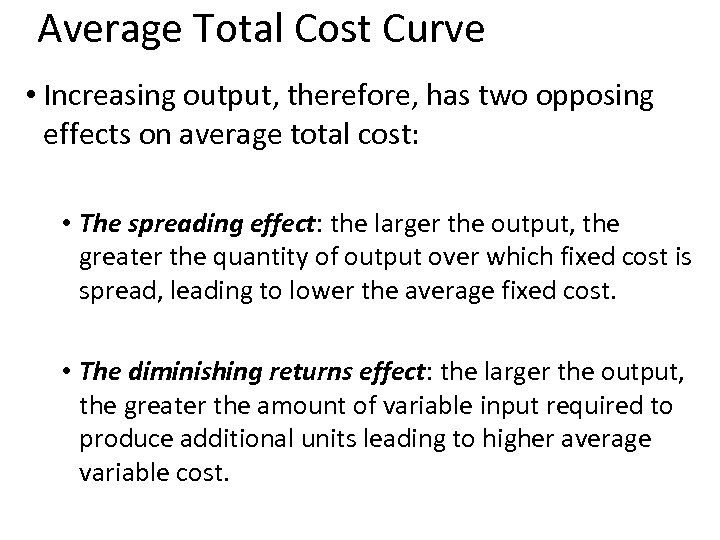Average Total Cost Curve • Increasing output, therefore, has two opposing effects on average total cost: • The spreading effect: the larger the output, the greater the quantity of output over which fixed cost is spread, leading to lower the average fixed cost. • The diminishing returns effect: the larger the output, the greater the amount of variable input required to produce additional units leading to higher average variable cost.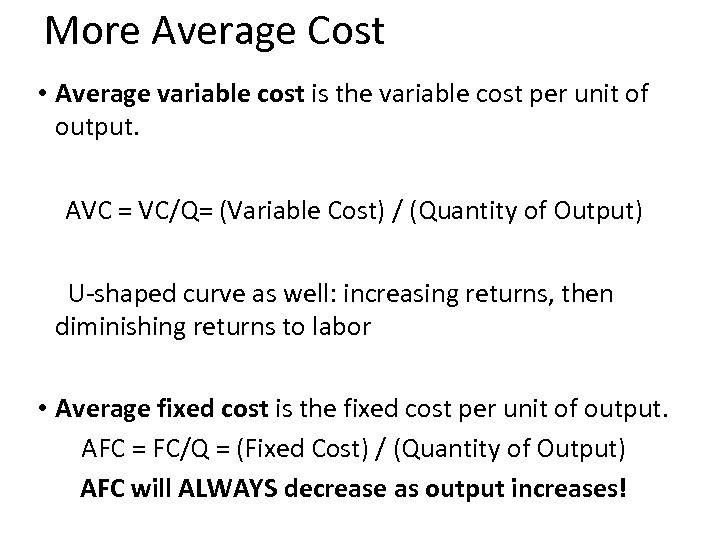More Average Cost • Average variable cost is the variable cost per unit of output. AVC = VC/Q= (Variable Cost) / (Quantity of Output) U-shaped curve as well: increasing returns, then diminishing returns to labor • Average fixed cost is the fixed cost per unit of output. AFC = FC/Q = (Fixed Cost) / (Quantity of Output) AFC will ALWAYS decrease as output increases!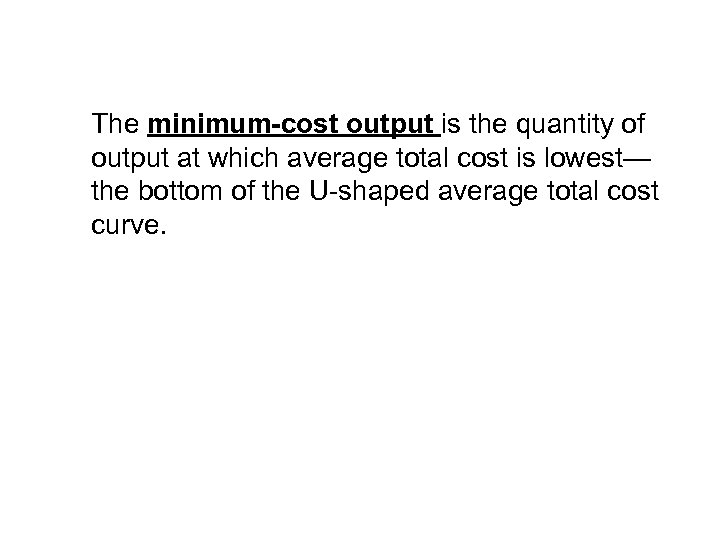The minimum-cost output is the quantity of output at which average total cost is lowest— the bottom of the U-shaped average total cost curve.Have your completed graphs on your desk.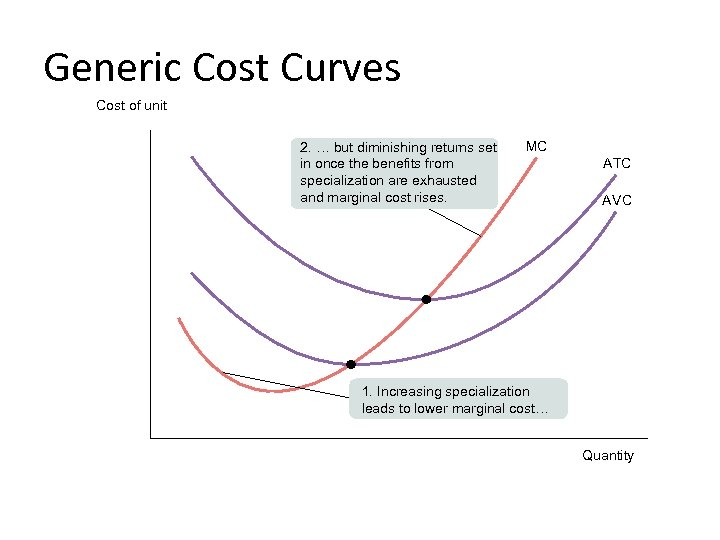Generic Cost Curves Cost of unit 2. … but diminishing returns set in once the benefits from specialization are exhausted and marginal cost rises. MC ATC AVC 1. Increasing specialization leads to lower marginal cost… Quantity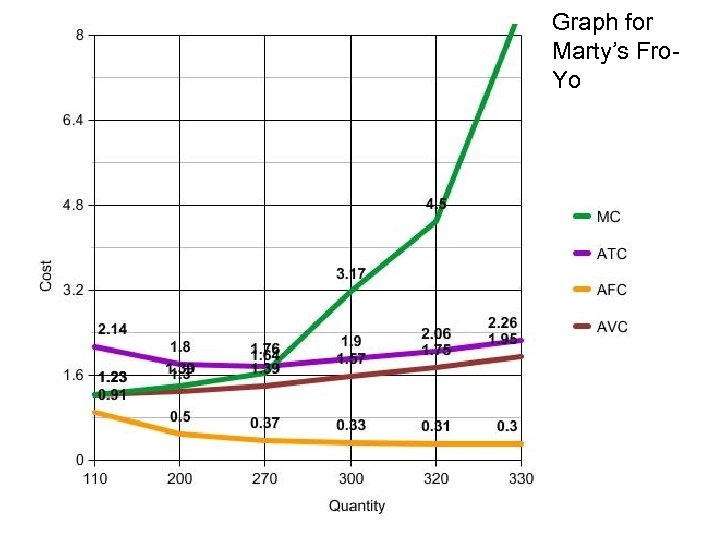Graph for Marty’s Fro. Yo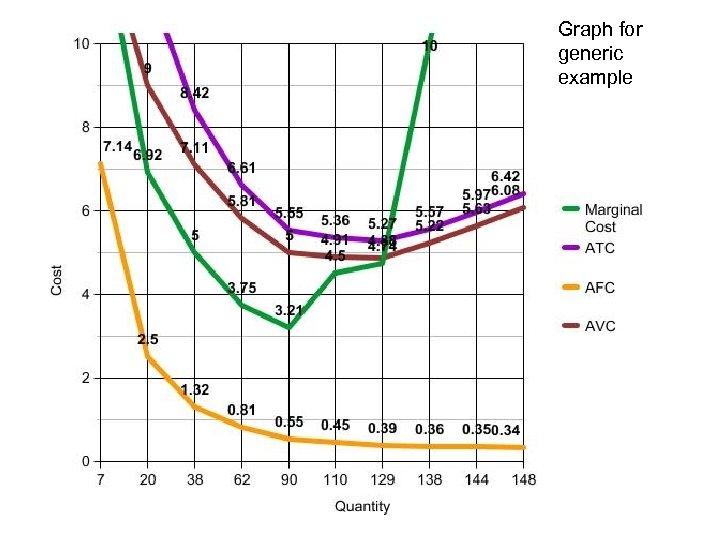Graph for generic example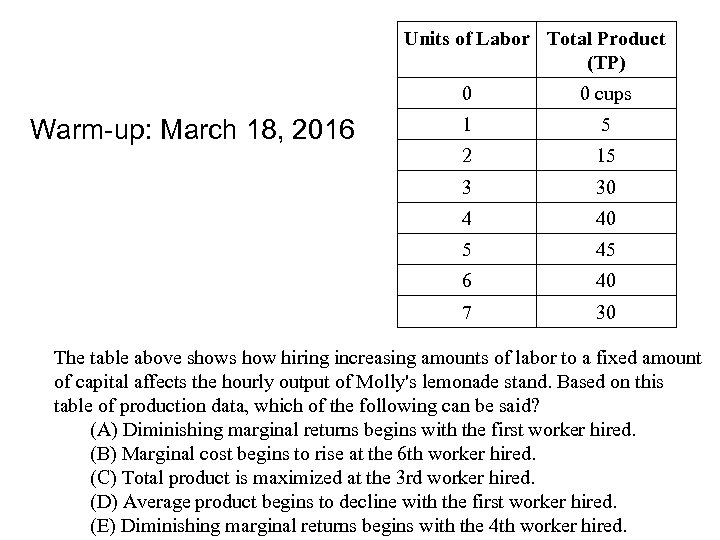Units of Labor Total Product (TP) 0 1 5 2 15 3 30 4 40 5 45 6 40 7 Warm-up: March 18, 2016 0 cups 30 The table above shows how hiring increasing amounts of labor to a fixed amount of capital affects the hourly output of Molly's lemonade stand. Based on this table of production data, which of the following can be said? (A) Diminishing marginal returns begins with the first worker hired. (B) Marginal cost begins to rise at the 6 th worker hired. (C) Total product is maximized at the 3 rd worker hired. (D) Average product begins to decline with the first worker hired. (E) Diminishing marginal returns begins with the 4 th worker hired.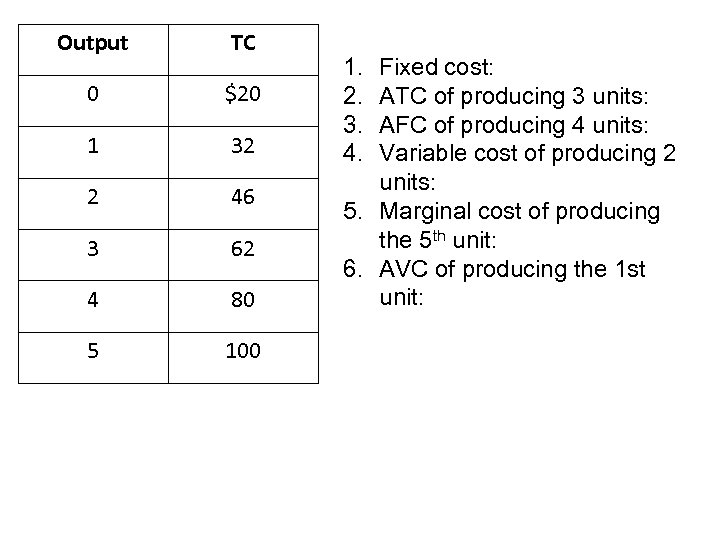Output TC 0 \$20 1 32 2 46 3 62 4 80 5 100 1. 2. 3. 4. Fixed cost: ATC of producing 3 units: AFC of producing 4 units: Variable cost of producing 2 units: 5. Marginal cost of producing the 5 th unit: 6. AVC of producing the 1 st unit: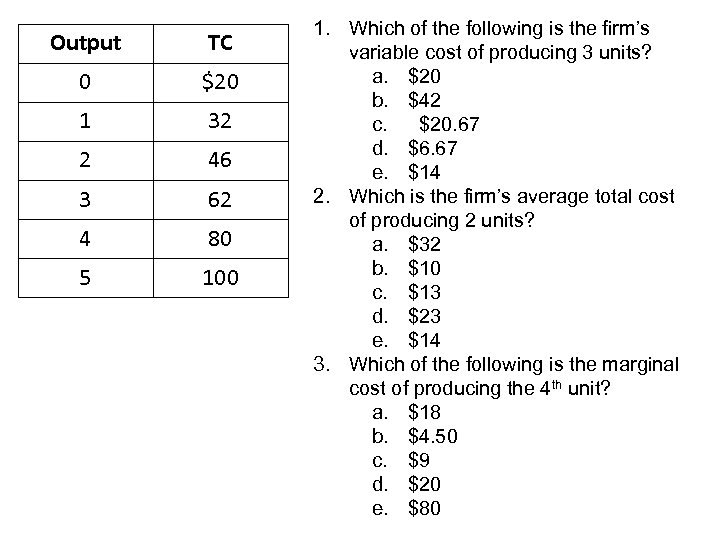Output TC 0 \$20 1 32 2 46 3 62 4 80 5 100 1. Which of the following is the firm’s variable cost of producing 3 units? a. \$20 b. \$42 c. \$20. 67 d. \$6. 67 e. \$14 2. Which is the firm’s average total cost of producing 2 units? a. \$32 b. \$10 c. \$13 d. \$23 e. \$14 3. Which of the following is the marginal cost of producing the 4 th unit? a. \$18 b. \$4. 50 c. \$9 d. \$20 e. \$80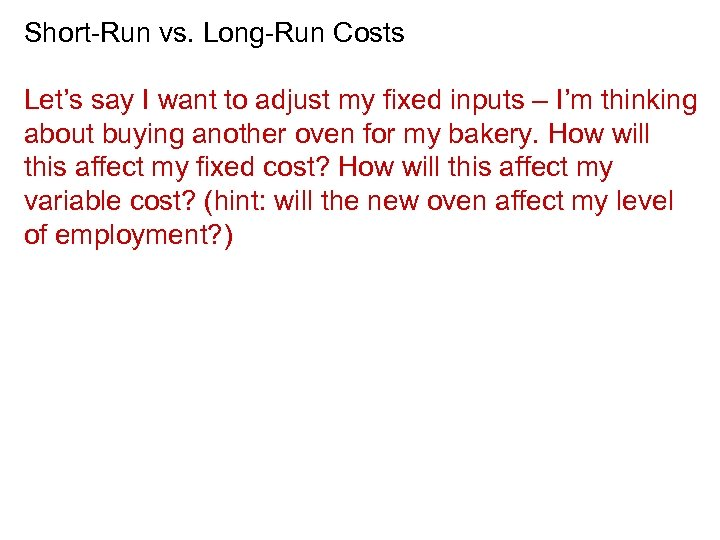Short-Run vs. Long-Run Costs Let’s say I want to adjust my fixed inputs – I’m thinking about buying another oven for my bakery. How will this affect my fixed cost? How will this affect my variable cost? (hint: will the new oven affect my level of employment? )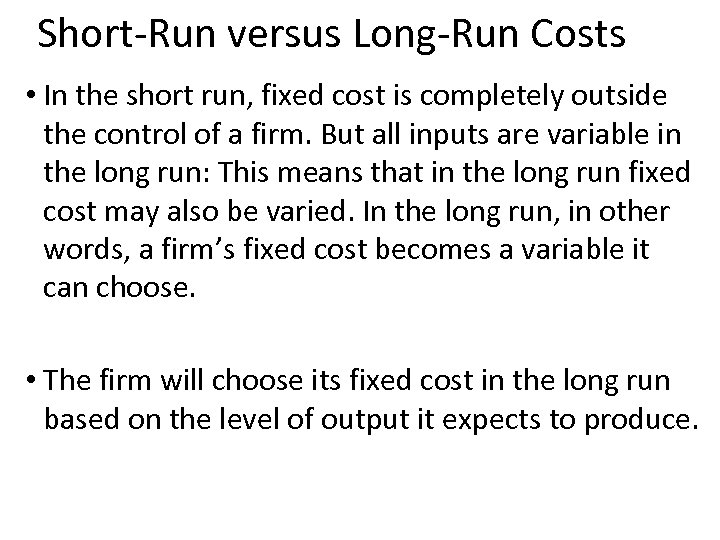Short-Run versus Long-Run Costs • In the short run, fixed cost is completely outside the control of a firm. But all inputs are variable in the long run: This means that in the long run fixed cost may also be varied. In the long run, in other words, a firm’s fixed cost becomes a variable it can choose. • The firm will choose its fixed cost in the long run based on the level of output it expects to produce.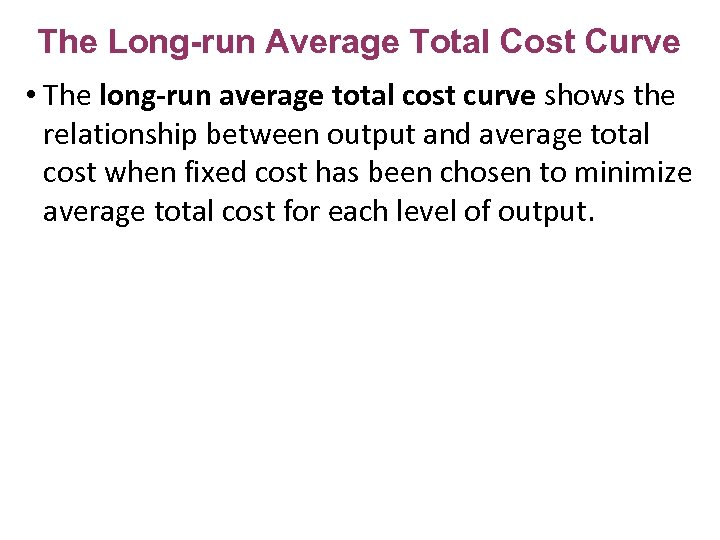The Long-run Average Total Cost Curve • The long-run average total cost curve shows the relationship between output and average total cost when fixed cost has been chosen to minimize average total cost for each level of output.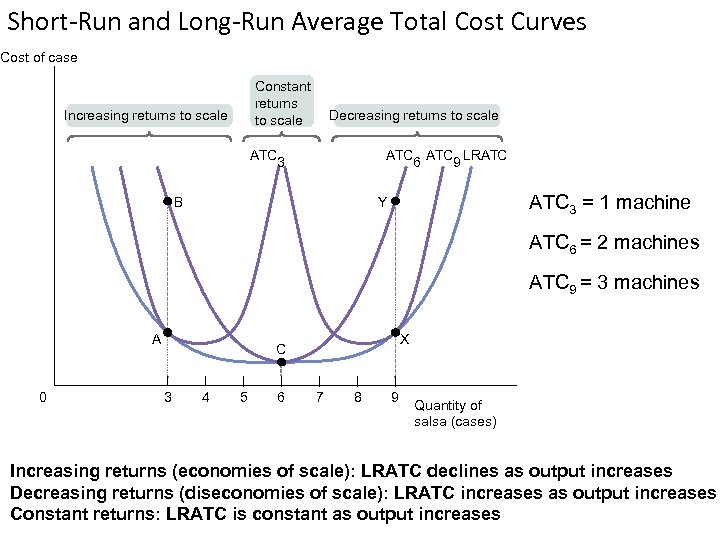Short-Run and Long-Run Average Total Cost Curves Cost of case Constant returns to scale Increasing returns to scale ATC Decreasing returns to scale ATC LRATC 6 9 3 B ATC 3 = 1 machine Y ATC 6 = 2 machines ATC 9 = 3 machines A 0 X C 3 4 5 6 7 8 9 Quantity of salsa (cases) Increasing returns (economies of scale): LRATC declines as output increases Decreasing returns (diseconomies of scale): LRATC increases as output increases Constant returns: LRATC is constant as output increases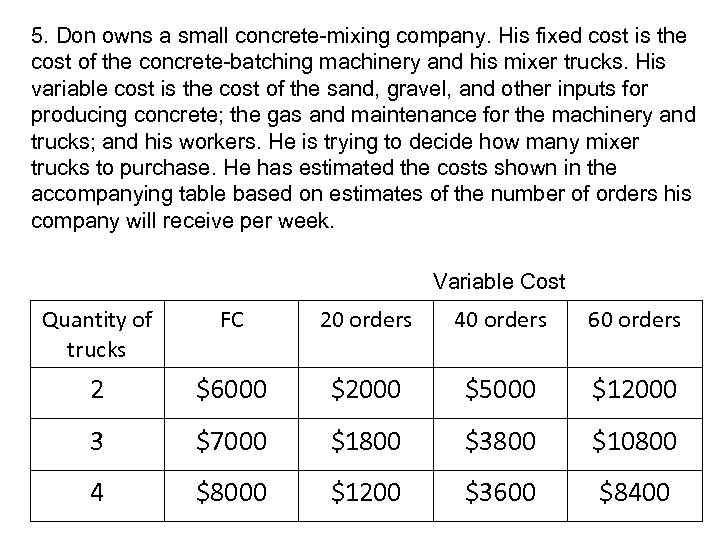5. Don owns a small concrete-mixing company. His fixed cost is the cost of the concrete-batching machinery and his mixer trucks. His variable cost is the cost of the sand, gravel, and other inputs for producing concrete; the gas and maintenance for the machinery and trucks; and his workers. He is trying to decide how many mixer trucks to purchase. He has estimated the costs shown in the accompanying table based on estimates of the number of orders his company will receive per week. Variable Cost Quantity of trucks FC 20 orders 40 orders 60 orders 2 \$6000 \$2000 \$5000 \$12000 3 \$7000 \$1800 \$3800 \$10800 4 \$8000 \$1200 \$3600 \$8400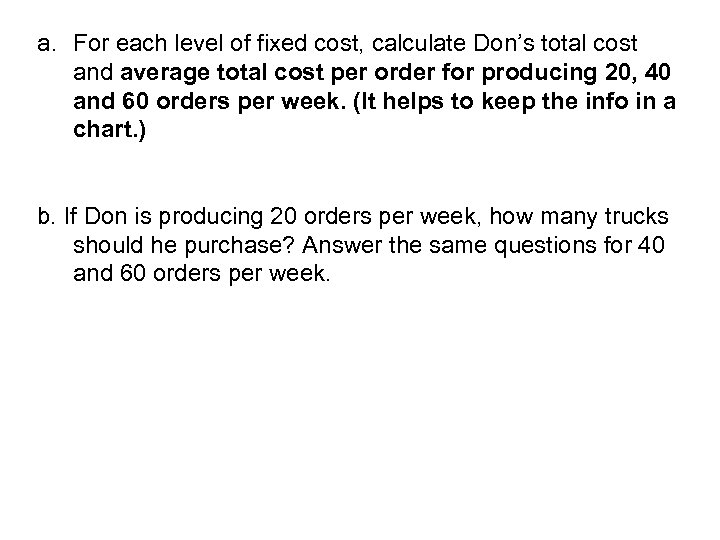a. For each level of fixed cost, calculate Don’s total cost and average total cost per order for producing 20, 40 and 60 orders per week. (It helps to keep the info in a chart. ) b. If Don is producing 20 orders per week, how many trucks should he purchase? Answer the same questions for 40 and 60 orders per week.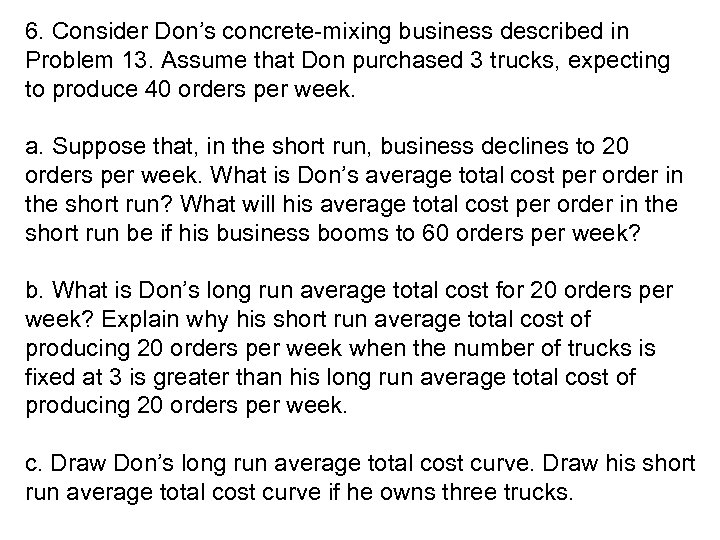6. Consider Don’s concrete-mixing business described in Problem 13. Assume that Don purchased 3 trucks, expecting to produce 40 orders per week. a. Suppose that, in the short run, business declines to 20 orders per week. What is Don’s average total cost per order in the short run? What will his average total cost per order in the short run be if his business booms to 60 orders per week? b. What is Don’s long run average total cost for 20 orders per week? Explain why his short run average total cost of producing 20 orders per week when the number of trucks is fixed at 3 is greater than his long run average total cost of producing 20 orders per week. c. Draw Don’s long run average total cost curve. Draw his short run average total cost curve if he owns three trucks.